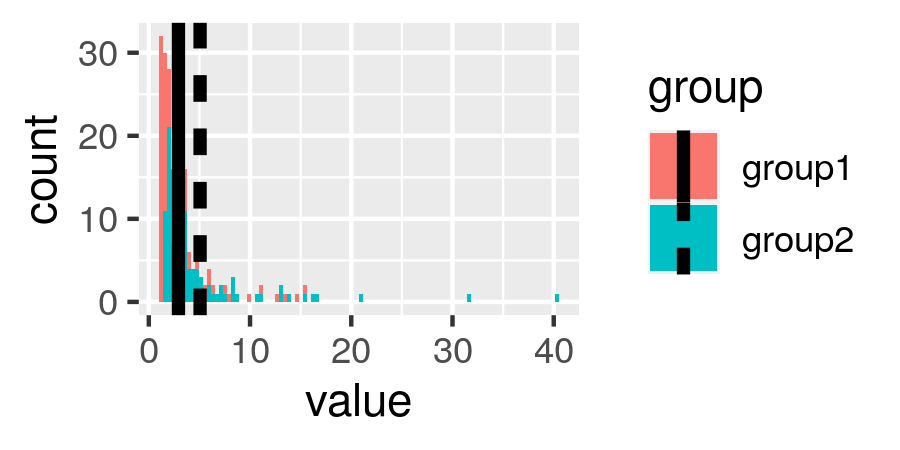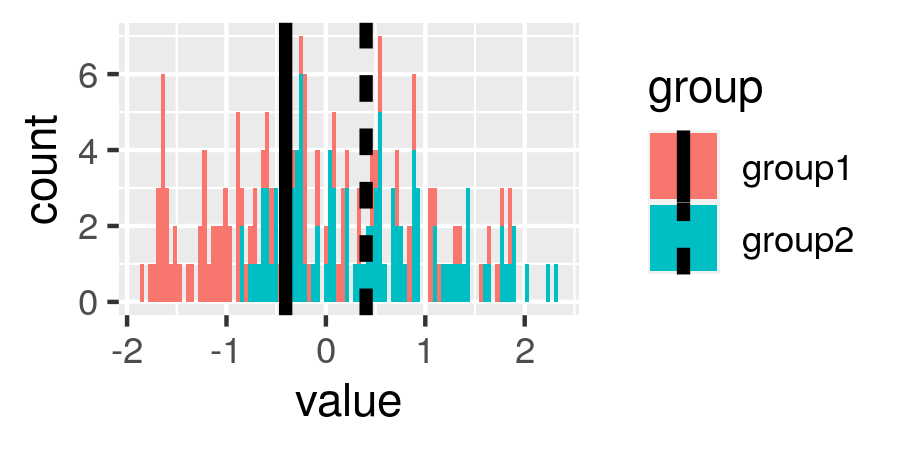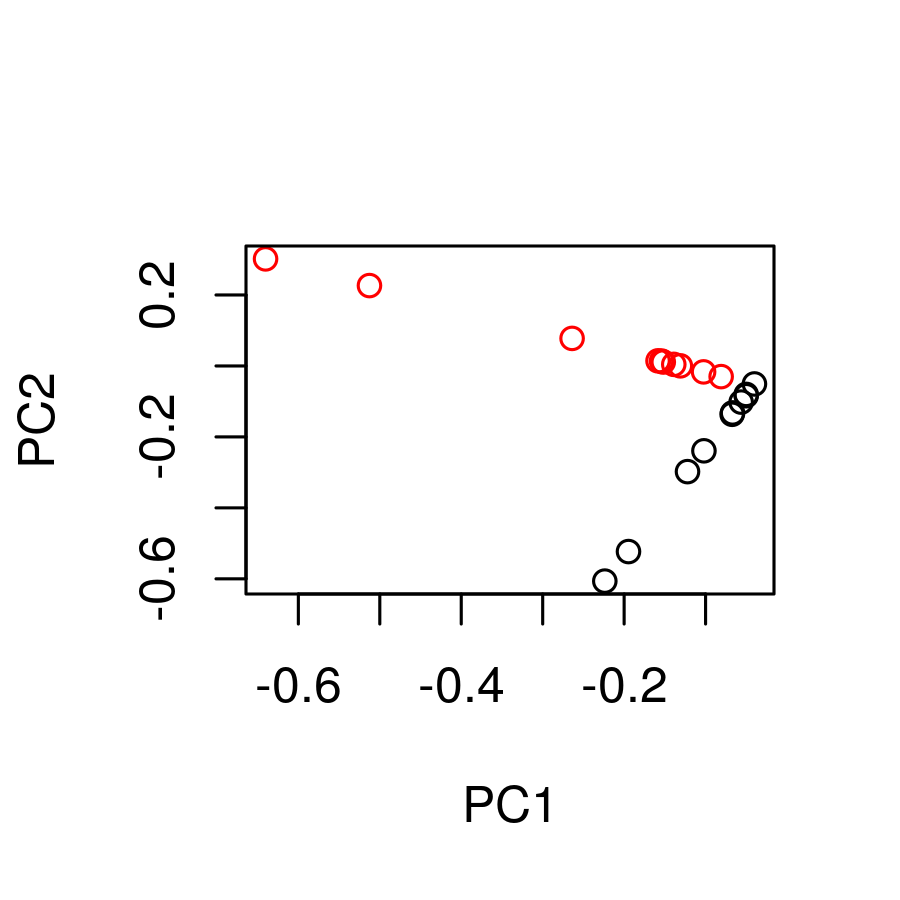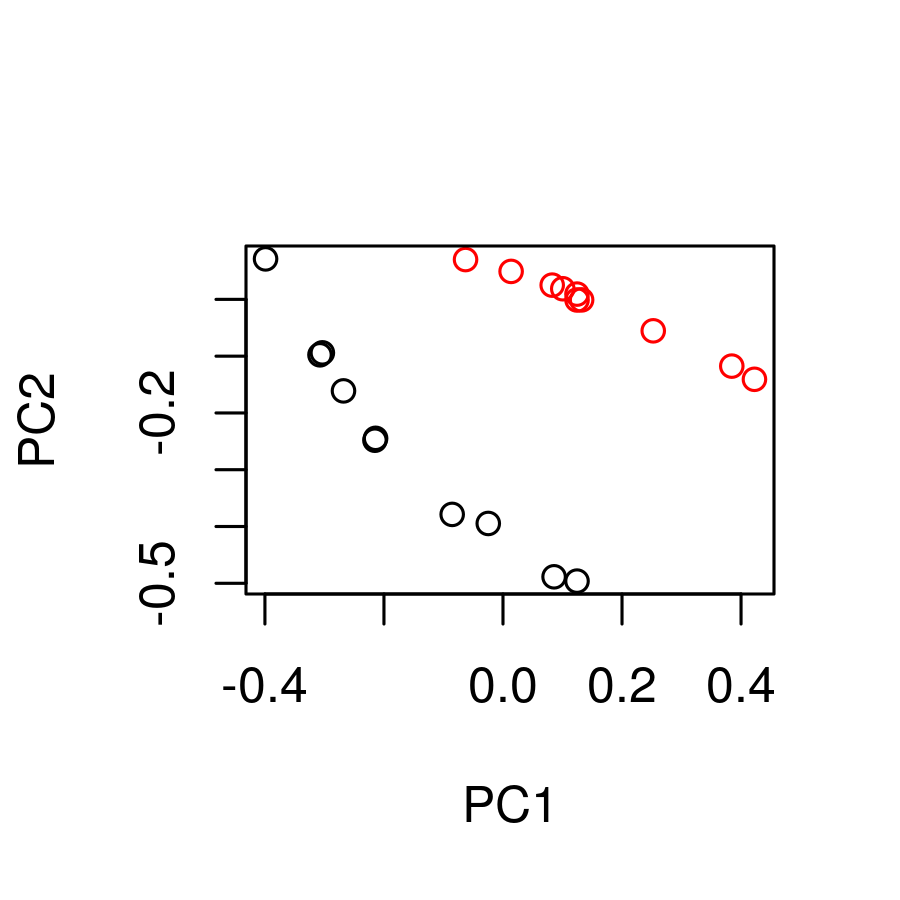# Rescaling Data

## RR overview

The Robust re-scaling transformation (RR) is a transformation the help reveal latent structure in data. It uses three steps to transform the data:

1. Gaussianize the data with a consensus box-cox-like transformation
2. z-score Transform the data using robust estimates of the mean and s.d.
3. remove extreme outliers from the data setting them to ‘NA’

The sequence of these transformations helps focus classic statistical analyses on consequential variance in the data rather than having the analyses be dominated by variation resulting from measurement scale or outliers.

The input to RR is a matrix or data.frame, the output is a matrix or data.frame of the same size, but with re-scaled values. RR works best when the columns of the data measure the features on similar scales (e.g. RNA-seq or microarray genomic data) and not data where the columns are fundamentally different-scale features.

## Applying RR

In this vignette we’ll look at how to use rrscale to re-scale data and help discover latent effects.

First we are going to generate data that is the concatenation of two log-normal groups to simulate data with groups. We do this by taking the outer product between two i.i.d. log-normal vectors, creating group 1 as:

set.seed(919)
u1 = rlnorm(10)
v1 = rlnorm(10)
Y1 = u1%*%t(v1)

and similarly group 2 as

u2 = rlnorm(10)
v2 = rlnorm(10)
Y2 = .5+u2%*%t(v2)

and then we concatenate these together to make a full data matrix (adding some noise)

Y_nn = rbind(Y1,Y2)
Y = Y_nn + array(rlnorm(prod(dim(Y_nn)),0,.05),dim(Y_nn))

Thus our data contains two groups, but notice that its difficult to tell the groups apart from a simple histogram:

library('reshape2')
library('ggplot2')
group = factor(rep(c(1,2),each=nrow(Y)/2))
levels(group) = c("group1","group2")
mY = melt(data.frame(Y,group),id.vars="group")
ggplot(data=mY,mapping=aes(x=value,fill=group))+geom_histogram(bins=100)+geom_vline(data=aggregate(value~group,data=mY,mean),mapping=aes(xintercept=value,linetype=group),size=1.5)Indeed if we look a t-test between the row means across groups we see no difference

t.test(rowMeans(Y)[group=="group1"],rowMeans(Y)[group=="group2"])
##
##  Welch Two Sample t-test
##
## data:  rowMeans(Y)[group == "group1"] and rowMeans(Y)[group == "group2"]
## t = -1.6241, df = 13.639, p-value = 0.1272
## alternative hypothesis: true difference in means is not equal to 0
## 95 percent confidence interval:
##  -4.9623864  0.6916029
## sample estimates:
## mean of x mean of y
##  2.930059  5.065451

Let’s try this after transforming the data with rrscale:

library('rrscale')
scl = rrscale(Y)

after running this we get an estimated transformation to help recover the latent group effect. The element “T_name” tells us that the best transformation is a box-cox-like transformation

scl$T_name ##  "box_cox_negative" and the element “par_hat” tells us the optimal value for the parameter to this transformation: scl$par_hat
##  -0.5206409

we can grab the pre-computed RR transformation from the call to rrscale

trans_Y = scl$RR or we can use the returned “rr_fn” to calcluate this transformation, they are identical trans_Y2 = scl$rr_fn(Y)
all(trans_Y2==trans_Y,na.rm=TRUE)
##  TRUE

Notice that if we plot the transformed Y we see that the group difference is easier to see:

tmY = melt(data.frame(trans_Y,group),id.vars="group")
ggplot(data=tmY,mapping=aes(x=value,fill=group))+geom_histogram(bins=100)+geom_vline(data=aggregate(value~group,data=tmY,mean),mapping=aes(xintercept=value,linetype=group),size=1.5)indeed the t-test is now significant

t.test(rowMeans(trans_Y)[group=="group1"],rowMeans(trans_Y)[group=="group2"])
##
##  Welch Two Sample t-test
##
## data:  rowMeans(trans_Y)[group == "group1"] and rowMeans(trans_Y)[group == "group2"]
## t = -2.7412, df = 16.971, p-value = 0.01394
## alternative hypothesis: true difference in means is not equal to 0
## 95 percent confidence interval:
##  -1.432490 -0.186342
## sample estimates:
##  mean of x  mean of y
## -0.4046867  0.4047291

If we plot he first two PCs for the transformed and un-transformed data we can see the group difference much better after transformation:

plot(svdc(Y)$u[,1:2],col=group,xlab="PC1",ylab="PC2")plot(svdc(trans_Y)$u[,1:2],col=group,xlab="PC1",ylab="PC2")Here we are using the “svdc” function from the rrscale package which calculates ’completed" right and left singular vectors in the presence of missing values. We can also look at the canonical correlation between the group and the first two PCS for the transformed and untransformed data:

cancor(model.matrix(~1+group),svdc(Y)$u[,1:2]) ##$cor
##  0.7074969
##
## $xcoef ## [,1] ## groupgroup2 0.4472136 ## ##$ycoef
##            [,1]      [,2]
## [1,] -0.3962615 1.6031726
## [2,]  0.9192846 0.7643497
##
## $xcenter ## (Intercept) groupgroup2 ## 1.0 0.5 ## ##$ycenter
##  -0.16540613 -0.08246504
cancor(model.matrix(~1+group),svdc(trans_Y)$u[,1:2]) ##$cor
##  0.9776598
##
## $xcoef ## [,1] ## groupgroup2 0.4472136 ## ##$ycoef
##          [,1]       [,2]
## [1,] 0.731373 -0.6820391
## [2,] 0.856082  0.9079851
##
## $xcenter ## (Intercept) groupgroup2 ## 1.0 0.5 ## ##$ycenter
##  -0.001640215 -0.133762712

and we can see that it is much higher for the transformed data signifiying these principal components capture the latent group structure better.